### 14.3.1.2 Sampling theory

Section 14.2.2 already covered some of the sampling issues. There are at least two continuous spaces:, and the time interval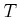. In most cases, the action space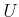is also continuous. Each continuous space must be sampled in some way. In the limit, it is important that any sample sequence is dense in the space on which sampling occurs. This was required for the resolution completeness concepts of Section 14.2.2.

Sampling ofandcan be performed by directly using the random or deterministic methods of Section 5.2. Time is just an interval of, andis typically expressed as a convex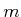-dimensional subset of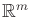. For example,is often an axis-aligned rectangular subset of.

Some planning methods may require sampling on. The definitions of discrepancy and dispersion from Section 5.2 can be easily adapted to any measure space and metric space, respectively. Even though it may be straightforward to define a good criterion, generating samples that optimize the criterion may be difficult or impossible.

A convenient way to avoid this problem is to work in a coordinate neighborhood of. This makes the manifold appear as an-dimensional region in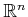, which in many cases is rectangular. This enables the sampling concepts of Section 5.2 to be applied in a straightforward manner. While this is the most straightforward approach, the sampling quality depends on the particular parameterization used to define the coordinate neighborhood. Note that when working with a coordinate neighborhood (for example, by imagining thatis a cube), appropriate identifications must be taken into account.

Steven M LaValle 2020-08-14